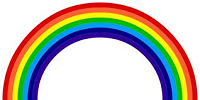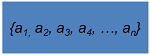# Finite Sequence: Definition & Examples

An error occurred trying to load this video.

Try refreshing the page, or contact customer support.

Coming up next: Geometric Series: Formula& Example

### You're on a roll. Keep up the good work!

Replay
Your next lesson will play in 10 seconds
• 0:00 What Is a Finite Sequence?
• 1:20 Nomenclature
• 1:52 Examples
• 2:42 Finding Patterns
• 5:07 Non-Examples
• 5:38 Lesson Summary

Want to watch this again later?

Timeline
Autoplay
Autoplay
Speed

#### Recommended Lessons and Courses for You

Lesson Transcript
Instructor: David Liano
After completing this lesson, you will be able to identify a finite sequence and different types of finite sequences. You will also be able to distinguish mathematical patterns of finite sequences.

## What Is a Finite Sequence?

If something is finite, then it has a limit or is bounded. Suppose you wanted to list the colors of the rainbow. It is generally acknowledged that there are seven colors of the rainbow. You could show the colors in set notation as follows:

red, orange, yellow, green, blue, indigo, violetThe list of colors stops at seven, so the colors of the rainbow are a finite set.

In mathematics, a sequence is usually meant to be a progression of numbers with a clear starting point. Some sequences also stop at a certain number. In other words, they have a first term and a last term, and all the terms follow a specific order. This type of sequence is called a finite sequence. Let's go back to the rainbow example. Notice that the colors are listed as they appear in the rainbow, from the topmost down.

Now, let's look at a mathematical example. The first five positive odd numbers are an example of a finite sequence; it stops at the number 9:

1, 3, 5, 7, 9

The elements of a sequence are not an arbitrary list of numbers. In other words, they are not listed randomly, but follow a specific order. Often, finite sequences follow a specific mathematical pattern that can be represented by a general rule that can be displayed in algebraic terms or in words.

## Nomenclature

In a finite sequence, there is a first term, second term, and so on until the last term. The letter n often represents the total number of terms in a finite sequence. The first term of a finite sequence can be represented by a(1), the second term by a(2), and so on. The number in parentheses next to the a is usually written as a subscript, but parentheses will be used at times in this lesson.

This figure illustrates this nomenclature.## Examples

There are many different types of finite sequences, but we will stay within the realm of mathematics. An example of a finite sequence is the prime numbers less than 40 as shown below:

2, 3, 5, 7, 11, 13, 17, 19, 23, 29, 31, 37

Another example is the natural numbers less than and equal to 100. It would be cumbersome to write out all of the terms in this finite sequence, so we can show it as follows:

1, 2, 3, 4, 5, …, 100

The ellipsis mark tells us that the pattern exhibited in the first five terms shown will continue until the last term of 100. There could be other sequences that start off the same way and end in 100 but that are not the natural numbers less than and equal to 100. In that case, it would be prudent to write the sequence sufficiently enough so that the reader understands the pattern.

## Finding Patterns

Let's look at some finite sequences and identify any patterns.

#### Example 1

A sequence in which all pairs of successive terms have a common difference is called an arithmetic finite sequence. Find the common difference in the following arithmetic finite sequence:

2, 7, 12, 17, …, 47

The first four terms of the sequence show that the common difference is 5. In other words, we can add 5 to any term in the sequence to get the next term in the sequence.

To unlock this lesson you must be a Study.com Member.

### Register to view this lesson

Are you a student or a teacher?

### Unlock Your Education

#### See for yourself why 30 million people use Study.com

##### Become a Study.com member and start learning now.
Back
What teachers are saying about Study.com

### Earning College Credit

Did you know… We have over 160 college courses that prepare you to earn credit by exam that is accepted by over 1,500 colleges and universities. You can test out of the first two years of college and save thousands off your degree. Anyone can earn credit-by-exam regardless of age or education level.